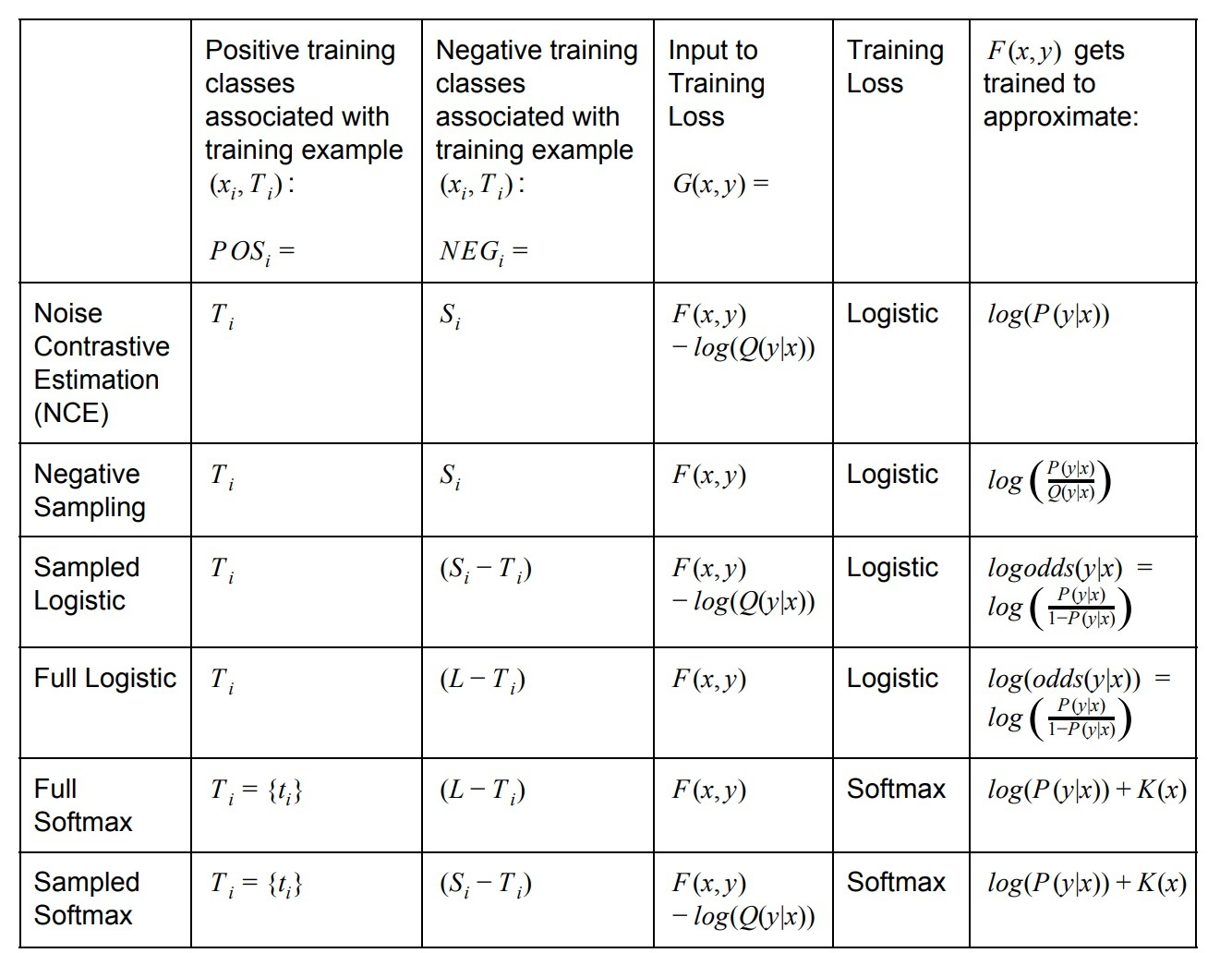# 1.介绍

## 1.1 F(x,y)

“穷举（Exhaustive）”训练法，比如：softmax和logistic regression需要我们为每个训练样本，对于每个类$y \in L$去计算F(x,y)。当$\mid L \mid$很大时，计算开销会很大。

## 1.2 $T_i$、$C_i$、$S_i$

• target classes（正样本）: $T_i$
• candidate classes（候选样本）: $C_i$
• randomly chosen sample of classes（负样本）: $S_i$

“候选采样（Candidate Sampling）”训练法，涉及到构建这样一个训练任务：对于每个训练样本$(x_i, T_i)$，我们只需要为候选类（candidate classes）$C_i \subset L$评估F(x,y)。通常，候选集合$C_i$是target classes和随机选中抽样的classes(非正例) $S_i \subset L$的合集(union)。• $Q(y \mid x)$: 被定义为：给定context x，根据抽样算法在sampled classes的集合中得到class y的概率（或：expected count）。
• $K(x)$：是一个任意函数（arbitrary function），不依赖于候选类（candidate class）。由于softmax涉及到一个归一化（normalization），加上这种函数不会影响到计算概率。
• logistic training loss =： $\sum\limits_i (\sum\limits_{y \in POS_i} log(1+exp(-G(x,y)) + \sum\limits_{y \in NEG_i} log(1+exp(G(x_i,y)) )$

• softmax \ training \ loss =：
• NCE 和Negatvie Sampling可以泛化到$T_i$是一个multiset的情况。在这种情况中，$P(y \mid x)$表示在$T_i$中y的期望数（expected count）。相似的，NCE，Negative Sampling和Sampled Logistic可以泛化到$S_i$是一个multiset的情况。在这种情况下，$Q(y \mid x)$表示在$S_i$中y的期望数（expected count）。

# Sampled Softmax

[bayes]{https://math.stackexchange.com/questions/549887/bayes-theorem-with-multiple-random-variables}

$P(Z|X,Y) = P(Y,Z|X) P(X) / P(X,Y) = P(Y,Z|X) P(Y|X)$…(b)

#

sampled_candidates的元素会使用base分布被无放回投样（如果：unique=True），否则会使用有放回抽样。

## log_uniform_candidate_sampler

1
2
3
4
5
6
7
8
9
tf.random.log_uniform_candidate_sampler(
true_classes,
num_true,
num_sampled,
unique,
range_max,
seed=None,
name=None
)


sampled_candidates的elements会从基础分布进行无放回抽样（如果unique=True）或者有放回抽样（unique=False）。

# 参考

https://www.tensorflow.org/extras/candidate_sampling.pdf

Updated on